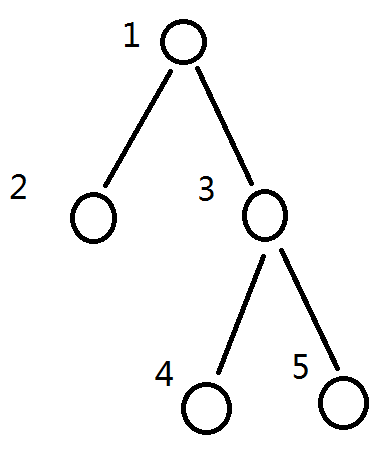# codeforces基础题——#362（div2）D

#### #362（Div 2） D

题目大意： 给你一棵n个节点的树(1 <= n <= 100000)， 根结点为1， 跑一遍dfs， 但每次dfs一个点的儿子时顺序是随机的， 然后问每个点在dfs序中出现位置的期望。可能的dfs序有 ： 1 2 3 4 5， 1 2 3 5 4， 1 3 4 5 2， 1 3 5 4 2


#include <cstdio>
#include <cstring>
using namespace std;
struct Edge{
int to, next;
}edge;
int head, num = -1;
void add_edge(int a, int b)
{
edge[++ num].to = b;
edge[num].next = head[a], head[a] = num;
}
double f, sz;
void dfs(int x)
{
sz[x] = 1;
for (int i = head[x]; i != -1; i = edge[i].next){
dfs(edge[i].to);
sz[x] += sz[edge[i].to];
}
}
void dp(int x)
{
for (int i = head[x]; i != -1; i = edge[i].next){
f[edge[i].to] = f[x] + 1 + (sz[x] - sz[edge[i].to] - 1) / 2;
dp(edge[i].to);
}
}
int main()
{
int n, x;
scanf("%d", &n);
memset(head, -1, sizeof(head));
for (int i = 2; i <= n; i ++){
scanf("%d", &x);
add_edge(x, i);
}
dfs(1); //printf("*");
f = 1.0; dp(1);
for (int i = 1; i <= n; i ++) printf("%.1lf ", f[i]);
printf("\n");

return 0;
}